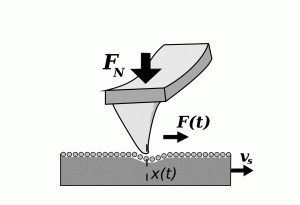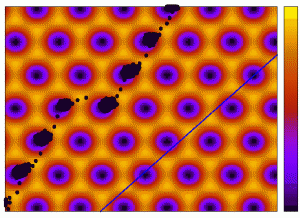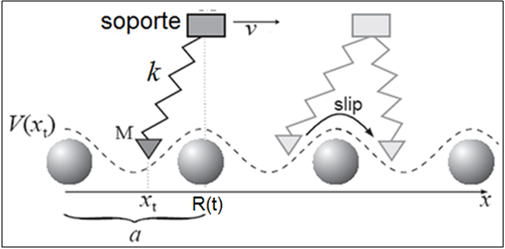# Friction at the nanoscale

People involved: Juan J. Mazo and Oscar Fajardo (PhD student)

Collaborations: Enrico Gnecco (IMDEA Nanociencia), Carlos Pina (UCM)Nanotribology is science that studies adhesion, friction, wear, and lubrication phenomena. The understanding of friction at nanoscale is a current important problem from both a fundamental and a technological perspective in wide ranges of length and time scales. On the one hand the understanding of friction at nanoscale is fundamental for the manipulation of nanoparticles and the miniaturization of moving devices as nano-electromechanical systems (NEMS), for instance. On the other hand friction is a complex nonlinear phenomenon of fundamental interest in many scientific areas. The development of new experimental techniques such as the force friction microscope and the surface force apparatus, experimental and theoretical studies of friction at the atomic scale have received a significant attention. Despite to the recent progress achieved in the last decades many of the fundamental problems in the field remain without answer it. In fact, we can say the more learn about friction, the more complex it seem. For instance, there not exists a theory to explain the most basic equation of friction, the Amontons’s law that describes phenomena known by Leonardo da Vinci 500 year ago: The lateral force of friction is proportional to the applied load.In general, we are interesting to study frictional phenomena at atomic scale by using nonlinear dynamics models like the well-known Prandtl-Tomlinson (PT) and numeric simulation. In this level of approximation, a tip of Atomic Force Microscopy (AFM) that is dragged over a surface in a typical nanotribology experimental setup is modeled as a point mass connect by a spring to a mobile support with constant velocity. This kind of approximation allows us to analyze the main properties of the system (the Force velocity and temperature curve, for instance) by including the most relevant degree of freedom (minimalistic model). This way to analyze the problem has shown describes many of the experimental results (for instance, stick-slip phenomenon which is believed to be the main mechanism of dissipation at atomic scale) and predicts new interesting outcome.

Recent research includes:

1. How tiny modification like small uncertainties or the presence of defects in the interaction effective potential affects the frictional behavior in the stick-slip regime.
2. Anisotropy effects and temperature on atomic friction
3. How the in- and out-plane actuation can modify the atomic friction.Publications